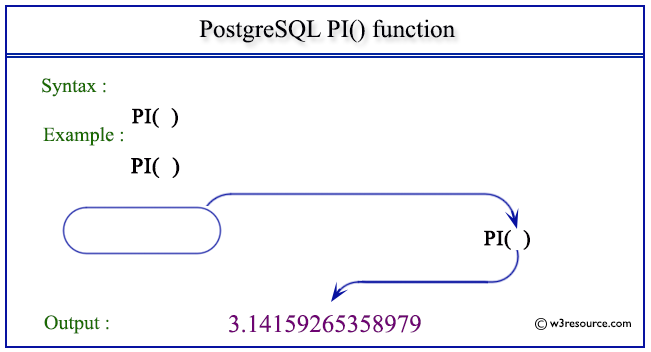# PostgreSQL PI() function

## PI() function

The PostgreSQL pi() function is used to return the constant value of pi.

Syntax:

```pi()
```

PostgreSQL Version: 9.3

Pictorial presentation of PostgreSQL PI() functionExample: PostgreSQL PI() function :

Code:

``````SELECT PI() AS "Value of PI";
```
```

Sample Output:

```    Value of PI
------------------
3.14159265358979
(1 row)
```

Previous: MOD function
Next: POWER function

﻿